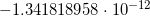# Importance of Multiprecision Computing

by on October 5, 2011

In this post we would like to show one of the numerous examples on why arbitrary precision computing is important. This particular example is taken from excellent paper “Why and how to use arbitrary precision” written by the main developers of MPFR: Vincent Lefèvre, Philippe Théveny, Paul Zimmermann in collaboration with Kaveh R. Ghazi.

Worth to note that without MPFR library it would be much more difficult to create Multiprecision Computing Toolbox or impossible at all. We are deeply grateful to the creators for their outstanding work.

Let’s compute following expression using standard floating-point arithmetic in MATLAB:

>> a = sin(1e22); >> b = log(17.1); >> c = exp(0.42); >> d = 173746*a + 94228*b - 78487*c d = 1.455191522836685e-011

Expression seems to be simple enough and result looks perfect with one little exception – it is completely wrong. Correct value is:.

The Multiprecision Computing Toolbox gives correct result (even using default accuracy of 20 decimal digits):

>> mp('173746*sin(1e22) + 94228*log(17.1) - 78487*exp(0.42)') -1.3418189560225439461e-12

For reasons why double arithmetic is not able to produce valid result please check original paper.